# The mathematical operators used in Google Sheets

Last updated Jul 09, 2022

You will need to perform a variety of mathematical operations when working with numbers in Google Sheets. Formulas are used to perform mathematical operations. Formulating your mathematical problem requires you to know how to use the Google sheet mathematical operators.

With Google Sheets, you can perform many mathematical operations, making it ideal for data analysis. This article will cover the mathematical operators that you can use if you are unfamiliar with the built-in routines.

The Common Operators in Google Sheets

Here are the common operators you can use to carry out mathematical operations.

The Subtraction operator (-):  Using this operator, we can subtract between two or more numbers in different cell references. In this case, we are subtracting A1 from B1.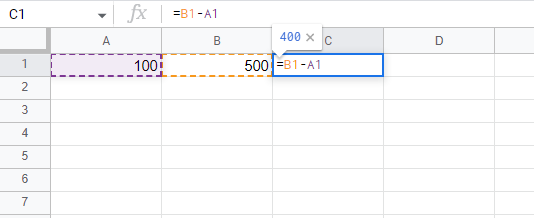Pressing enter on your keyboard will display the answer in cell C1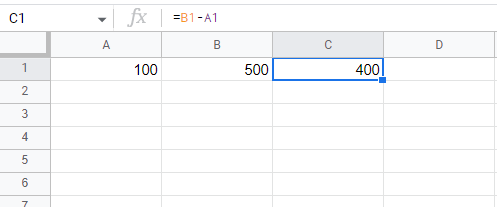The Addition operator (+): You can use this operator to add two or more numbers in two or more cell references. Here, we are adding A1 and B1.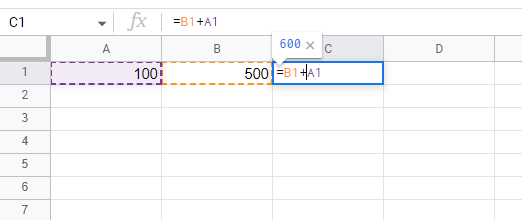If you press enter on your keyboard, the answer will appear in cell C1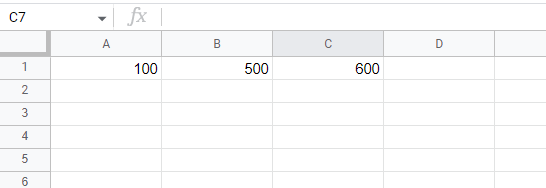The Multiplication (*) operator: By using this operator, you can multiply numbers across two or more cell references. Here, we are multiplying A1 by B2.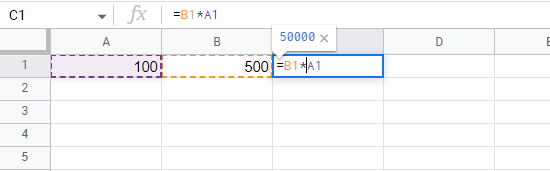Pressing enter on your keyboard now displays the answer in cell C1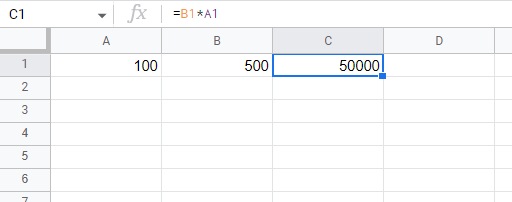The Division operation (/): By using this operator, you can divide numbers between two or more references. For this example, we will divide A1 by B2.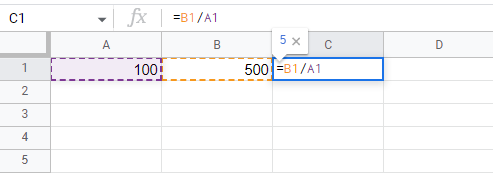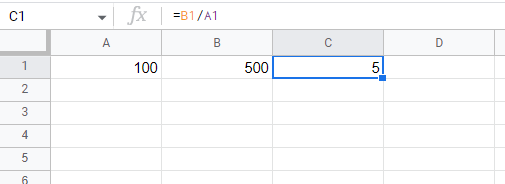Conclusion

In Google Sheets, conventional formula operators are used for addition (+), subtraction (-), asterisks (*), forward slashes (/), and carets () for exponents. Please note that every formula must begin with a Tweddle v Atkinson  EWHC

symbol (=). It is because the cell contains-or equals-the formula and its result

 Article Contributed By :61 Views
Projects By category
Categories